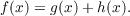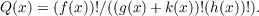#### Vol. 17, No. 2, 1966

 Download this articleFor screen For printingRecent Issues Vol. 323: 1 Vol. 322: 1  2 Vol. 321: 1  2 Vol. 320: 1  2 Vol. 319: 1  2 Vol. 318: 1  2 Vol. 317: 1  2 Vol. 316: 1  2Online Archive Volume: Issue:The Journal Subscriptions Editorial Board Officers Contacts Submission Guidelines Submission Form Policies for Authors ISSN: 1945-5844 (e-only) ISSN: 0030-8730 (print) Special Issues Author Index To Appear Other MSP Journals
A problem complementary to a problem of Erdős

### J. Chidambaraswamy

Vol. 17 (1966), No. 2, 227–234
##### Abstract

Let f(x), g(x), and h(x) be rational integer coefficient polynomials of positive degree and with positive leading coefficients and satisfying(1.1)

k(x) also being such a polynomial of degree 0, let(1.2)

Question 1: Is Q(x) integral for an infinity of integers x, at least when k(x) of degree zero, say k(x) = k(1)?

Question 2: Is Q(x) nonintegral for all sufficiently large integers x, at least when the degree of k(x) is 1? No general answer is known to both these questions. In this paper, we consider the question of existence of an infinity of integers x for which Q(x) is not an integer: in the context of question 1, we obtain certain conditions on the coefficients of g(x) and h(x) and k to ensure the existence of an infinity of integers x for which Q(x) is not an integer, and in the context of question 2, we prove Q(x) is nonintegral infinitely often.

Primary: 10.05
##### Milestones